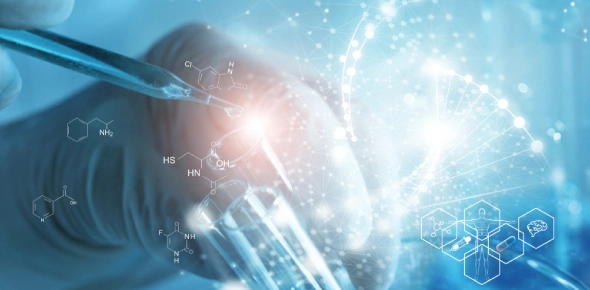# Prep For Biology Exam - Silke Morin

69 Questions | Attempts: 36
ShareSettingsQuiz for final exam for intro to bio - a&p

• 1.
Which off the following is the smallest metric prefix?
• A.

Milli

• B.

Hecto

• C.

Centi

• D.

Kilo

• 2.
The smallest bit of matter that has the properties of a specific element is an?
• A.

Atom

• B.

Electron

• C.

Molecule

• D.

Proton

• 3.
The subatomic particle that has a positive electrical charge is a?
• A.

Electron

• B.

Ion

• C.

Neutron

• D.

Proton

• 4.
The subatomic particle that has a negative charge is a?
• A.

Electron

• B.

Proton

• C.

Neutron

• D.

Ion

• 5.
The isotopes of an element are
• A.

Atoms of that element that have the same number of protons but different number of electrons

• B.

Atoms of that element that have the same number of protons but different number of neutrons

• 6.
Most atoms with 1 electron in the outermost shell tend to
• A.

Give up an electron to another atom that has a strong attraction to electrons

• B.

Take an electron from another atom so that it has an electron pair

• 7.
Identify the chemical reaction that shows decomposition
• A.

H2co3 - h+ & hco3

• B.

H + hco3 - h2co3

• 8.
When molecules that contain polar covalent bonds are close to each other, they form _____ between adjacent molecules
• A.

Hydrogen bonds

• B.

Carbon bonds

• 9.
Which property of water refers to the fact that it absorbs a lot of heat without changing its temperature?
• A.

• B.

Cohesion

• C.

High heat capcity

• D.

High heat vaporization

• 10.
Which property of water refers to the fact that water molecules are attracted to other water molecules?
• A.

Cohesion

• B.

• 11.
Which property of water refers to its ability to dissolve substances?
• A.

Solvency

• B.

Density

• C.

Surface tension

• D.

Capillary action

• 12.
Basic metric length for:length
• 13.
Basic metric length for:mass
• 14.
Basic metric length for:volume
• 15.
Convert:0.025 milligrams to grams
• 16.
Convert:57 kilometers to meters
• 17.
Which of the following is not an organic compound
• A.

Dna

• B.

Glucose

• C.

Water

• 18.
What do all organic compounds always have
• A.

Carbon

• B.

Hydrogen

• C.

Electrons

• D.

Protons

• 19.
What is a monomer of a lipid
• A.

Amino acid

• B.

Glycerol or fatty acid

• 20.
What is a monomer of a carb?
• A.

Nucleotide

• B.

Monosaccharide

• 21.
Which molecule functions as an energy carrier?
• A.

ATP

• B.

Carb

• 22.
The sum of all chemical reactions within a living cell is
• A.

Metabolism

• B.

Catabolism

• C.

Anabolism

• 23.
ATP is composed of
• A.

Ribose sugar

• B.

• C.

3 phosphate groups

• D.

1 phosphate group

• E.

2 phosephate groups

• 24.
What is the place whyere substrates fit onto the enzyme in such a way that they are oriented to react?
• A.

Active site

• B.

Coenzyme

• 25.
The basic structural and functional unit of living organisms is
• A.

Dna

• B.

Cell

• C.

Organic compoundBack to top
×

Wait!
Here's an interesting quiz for you.